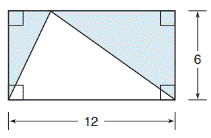Chapter 8.1, Problem 20E### Elementary Geometry for College St...

6th Edition
Daniel C. Alexander + 1 other
ISBN: 9781285195698

#### Solutions

Chapter
Section### Elementary Geometry for College St...

6th Edition
Daniel C. Alexander + 1 other
ISBN: 9781285195698
Textbook Problem
1 views

# In Exercises 19 to 22, find the area of the shaded region.To determine

To find:

The area of the shaded region.

Explanation

Finding the area of the figure is followed by identifying the figure and find area with the suitable geometry formulae.

Calculation:

Given,

Which represents the triangle inscribed in a rectangle.

The area of the shaded region(i.e.) A=lw12(bh)}=(A1)Area of a rectangle-(A2)Area of a triangle

Here,

Length of the rectangle (l)=12

Width of the rectangle (w)=6

### Still sussing out bartleby?

Check out a sample textbook solution.

See a sample solution

#### The Solution to Your Study Problems

Bartleby provides explanations to thousands of textbook problems written by our experts, many with advanced degrees!

Get Started

#### Find more solutions based on key concepts